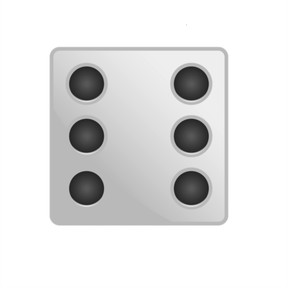Recognizing Dice to 6

# Recognizing Dice to 6

Recognizing Dice to 68,000 schools use Gynzy92,000 teachers use Gynzy1,600,000 students use Gynzy

## General

Students learn to recognize numbers to 6 on dice

K.CC.B.4

## Relevance

It is useful to recognize a number at once on dice so you don't need to count all the dots. You need this, for example, to play a game with dice and quickly know how many spaces you can move.

## Introduction

Discuss with students when you use dice. Then, refresh and repeat the numbers 1-6, making sure to ask what the numbers are called and what they look like.

## Development

Count the number of dots on the dice and write the number next to the dice. Then discuss all the sides of the dice with the students. During this time you show that the dots of dice never change, and you can always quickly see how many dots a side has.

To check that students recognize dice to 6 you can ask the following questions:
Are the dots always in the same place?
Which number fits this side of the dice?
Why is it useful to recognize the amount of dots on the dice?

## Guided Practice

The students practice which dice belongs to the number 3, which number belongs to dice with four dots, which number belongs to a dice with six dots, and which dice belongs to the numbers 3 and 5. Emphasize that they should be moving from counting the dots to recognizing the amount by the position the dots are in. Encourage the students to give answers as quickly as possible to minimize counting and maximize pattern recognition.

## Closing

Show the students sides of the dice and ask them to write down the number it represents. Following this, you can put the students in pairs and ask them to roll actual dice, with one student rolling and the other saying the shown number as quickly as possible.

## Teaching Tip

You can practice the numbers 1-6 with the students who don't recognize them yet.

## Instruction materials

Dice and possibly a board game.

### The online teaching platform for interactive whiteboards and displays in schools

• Save time building lessons

• Manage the classroom more efficiently

• Increase student engagement